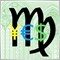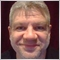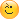# 1M data displayed in the data window for the 15M candle (MQL5)93

Hi,

I have started to look at MQL5, which is quite hard even if I have some informations about MQL4.

What I would like to do is to have in the data window (as an indicator), for each 15M candle, buffers of the 1M candles data that composed the 15M candle.

At the same time have the direction of the candle: 1 (open>close), 0 (close>open) for both the series of 1M candles and the 15M candle.

For example:

```Indicator retrieve data when the current 15M is closed

BUFFER N1 //--- this is the buffer of the EURUSD,M15 candle

Date    2017.09.29
Time    18:30 //--- let's suppose it is completed (so we can see it at 18:45)
Open    1.18013
High    1.18072
Low     1.18010
Close   1.18023
Direction  0  (open<close)

BUFFER N2 //--- this is the buffer of the EURUSD,M1 candle of the first minute of the 15M candle

Date    2017.09.29
Time    //--- 18:30:00 to 18:30:59
Open    1.18013
High    some value
Low     some value
Close   some value
Direction  depends on the close in this case

BUFFER N3 //--- this is the buffer of the EURUSD,M1 candle of the second minute of the 15M candle

Date    2017.09.29
Time    18:31:00 to 18:31:59
Open    some value
High    some value
Low     some value
Close   some value
Direction depens on the Open and close in this case

ecc.. till BUFFER N16 that close the 15 minutes.
```

So in this way I will be able to use the information collected by the indicator in a concise way.

I have tried something like this, using the CopyRates but I don't exactly have an idea and it is clearly wrong:

```#property indicator_chart_window
#property indicator_buffers 2

//--- indicator buffers
double    Candle[];
double    Candle15M[];

int OnInit()
{
//--- indicator buffers mapping
SetIndexBuffer(0,Candle,INDICATOR_DATA);
SetIndexBuffer(1,Candle15M,INDICATOR_DATA);

return(INIT_SUCCEEDED);
}

int OnCalculate(const int rates_total,
const int prev_calculated,
const datetime &time[],
const double &open[],
const double &high[],
const double &low[],
const double &close[],
const long &tick_volume[],
const long &volume[],
{
MqlRates rates[];
for (int i=(14);i>0;i--)
(
Candle = CopyRates(Symbol(),PERIOD_M1,0,14,rates);
Candle15M = CopyRates(Symbol(),0,0,0,rates);
)
return(rates_total);
}```37067

Buffer contains a single value for a bar. How do you suggest to pack OHLC (4 values) and direction (+1 value) into a single buffer element? If you want OHLC (4) + direction mark (1) for every M1 in M15 bar, then you need 15*5 buffers.42595

Stanislav Korotky:
Buffer contains a single value for a bar. How do you suggest to pack OHLC (4 values) and direction (+1 value) into a single buffer element? If you want OHLC (4) + direction mark (1) for every M1 in M15 bar, then you need 15*5 buffers.

No problem, limit is 51293

Yes lot's of data stored but I think that it won't exceed the limit. 15 bar of 1M needs 7 slot (date,time, open,close,low,high,direction) + 1 bar of 15M needs other 7 lslot = so total of 16*7 = 112 buffers.

To have it a little bit simpler, maybe 5 arrays, as below, that store the previous 1M minute data would be good + 1 array that stores the data for the 15M candle.

```double close_data[];
double open_data[];
double high_data[];
double low_data[];
double direction_data[];

int OnInit()
{
IndicatorBuffers(5);
SetIndexBuffer(0, close_data);
SetIndexBuffer(1, open_data);
SetIndexBuffer(2, high_data);
SetIndexBuffer(3, low_data);
SetIndexBuffer(4, direction_data);
ArraySetAsSeries(close_data,true);
ArraySetAsSeries(open_data,true);
ArraySetAsSeries(high_data,true);
ArraySetAsSeries(low_data,true);
ArraySetAsSeries(direction_data,true);
return(INIT_SUCCEEDED);
}

int OnCalculate(const int rates_total,
const int prev_calculated,
const datetime &time[],
const double &open[],
const double &high[],
const double &low[],
const double &close[],
const long &tick_volume[],
const long &volume[],
{
CopyClose(Symbol(),PERIOD_M1,1,15,close_data);
CopyOpen(Symbol(),PERIOD_M1,1,15,open_data);
CopyHigh(Symbol(),PERIOD_M1,1,15,high_data);
CopyLow(Symbol(),PERIOD_M1,1,15,low_data);
for (int i=1;i<=15;i++)
{
if (close_data[i]> open_data[i])
{
direction_data[i]=0;
}
if (close_data[i]<= open_data[i])
{
direction_data[i]=1;
}

}
return(0);
}```

I have tried to re-write it in mql4 but results are not good...37067

Alain Verleyen:

No problem, limit is 512

The problem was that OP did not reserve and use these buffers in the initial source code, while his explanation in the pseudo-code implied this.

Ok, if it's simplified to 5 buffers, and if I understand correctly, you need to copy only one M1 value into i-th bar on M15 for every OHLC+D slot. On i+1 bar you'd copy from another M1 bar corresponding to the next M15 bar and so on. Currently you copy M1 into M15 flatly, i.e. display M1 bars in M15 chart.